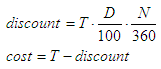# Discount Commercial Paper

This small exercise in Matlab calculates the discount amount and net cost of a discount commercial paper. You must provide the future value of the paper, the discount rate and the number of days to maturity.

The formulas used to calculate the discount and cost are as follows:where:
T = total future value
D = discount rate
N = number of days to maturity

We then create a Matlab function to calculate the formulas above:

function [d1, c] = commercial_paper(t,d,n)
d1 = t * d/100 * n/360;
c = t - d1;

We can create another script to test and drive the above m-file:

clc; clear; close all; format bank; format compact

t = input('Enter future value: ');
d = input(
'Enter discount rate: ');
n = input(
'Enter number of days to maturity: ');

[d, c] = commercial_paper(t, d, n)

Example:

SuperAcme Corporation purchases a \$625,000 comm. paper due in 60 days at 5.4%. What's the discount and cost?

We run our driving code and enter:

Enter future value: 625000
Enter discount rate: 5.4
Enter number of days to maturity: 60

The result is:

d = 5625.00
c = 619375.00

From 'Commercial Paper' to home

From 'Commercial Paper' to 'Finance Formulas'